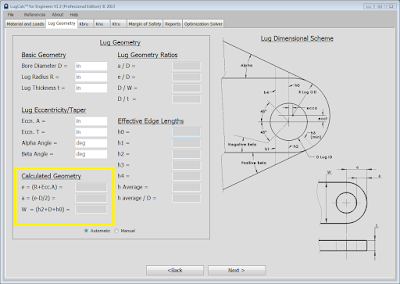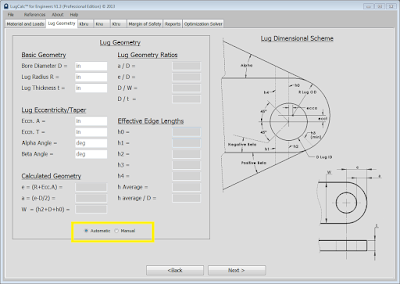# Calculated Lug Geometry

The following geometric dimensions are calculated from basic geometry and effective edge lengths:

e = (R + ecca) where ecca = axial eccentricity offset
a = (e – D / 2)
W = (h2 + D + h0)Calculated Geometry Input Fields

For straight lugs, these dimensions are easily obtained, lugs with taper and eccentric bore locations complicate calculation. LugCalc™ will calculate these values automatically for all scenarios. If the user desires to enter them manually, click the manual radio button at the bottom of the geometry window. In manual geometry mode, the user will be required to enter all the geometric values in the “Calculated Geometry” fields (nothing will be calculated automatically).Automatic/Manual Geometry Calculation Radio Button